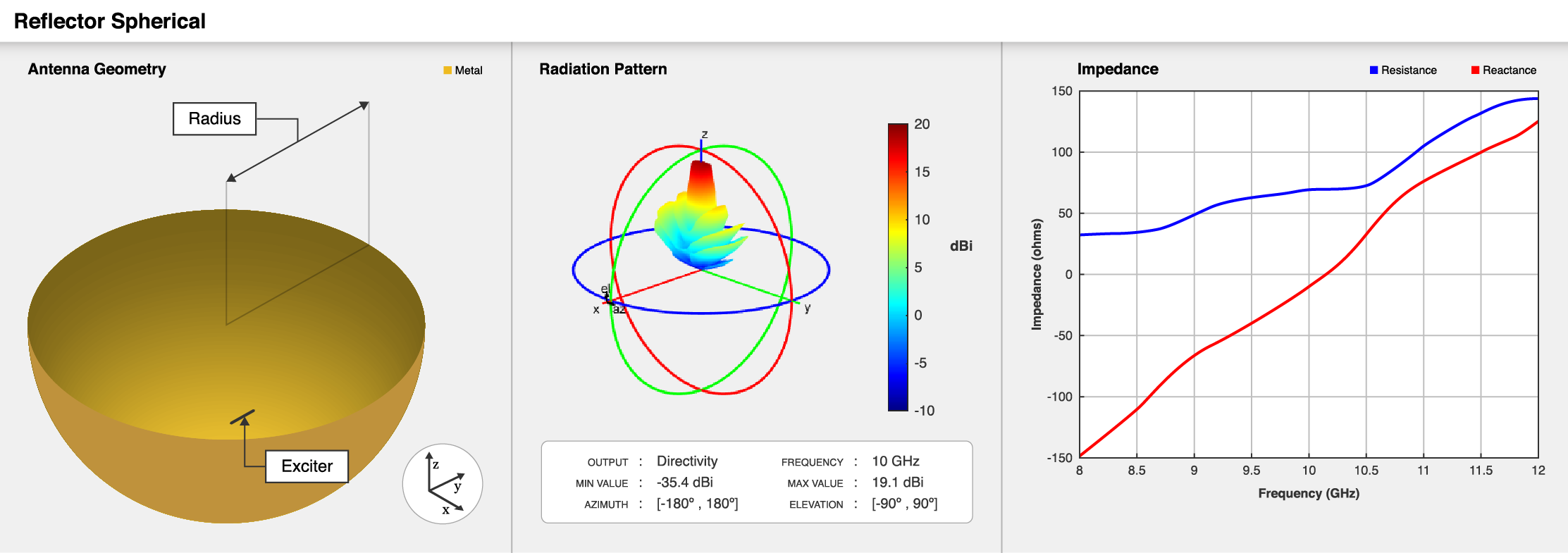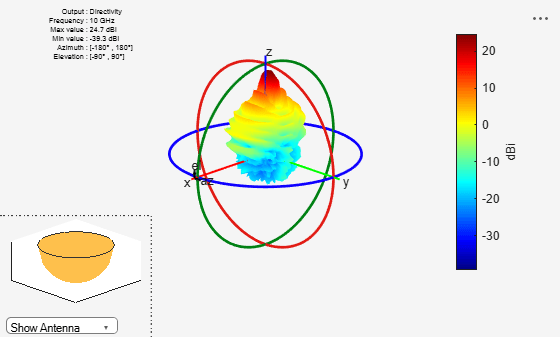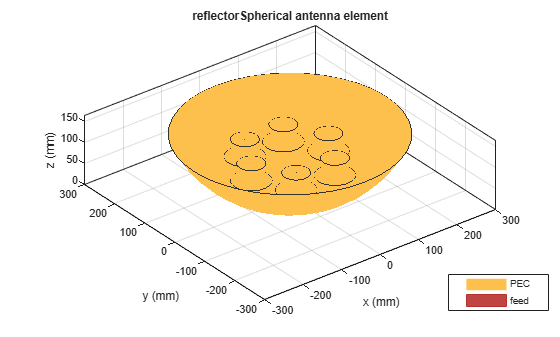# reflectorSpherical

Create spherical reflector-backed antenna

## Description

The `reflectorSpherical` antenna object creates a spherical reflector-backed antenna. The reflector in the spherical reflector-backed antenna is one-half the size of the sphere. The antenna is used in wide-angle scanning on account of its perfectly symmetrical geometric configuration.## Creation

### Syntax

``ant = reflectorSpherical``
``ant = reflectorSpherical(Name,Value)``

### Description

example

````ant = reflectorSpherical` creates a spherical reflector-backed antenna. The default antenna object has an exciter as a center-fed dipole located on the X-Y plane. The default antenna object dimensions are chosen for an operating frequency of 1 GHz.```

example

````ant = reflectorSpherical(Name,Value)` sets Properties using name-value pairs. For example, `reflectorSpherical('Radius',0.6)` sets the spherical reflector radius to 0.6 meters. You can specify multiple name-value pairs. Enclose each property name in quotes. Properties not specified retain their default values.```

## Properties

expand all

Antenna used as an exciter, specified as an `antenna` object or an `array` object.

Example: `'Exciter',dipole`

Example: ```ant.Exciter = dipole('Length',0.1409,'Width',0.02,'FeedOffset',0,'Tilt',90,'TiltAxis',[0 1 0])```

Example: ```ant.Exciter = linearArray('patchMicrostrip')```

Radius of the spherical reflector aperture along X and Y-axes, specified as a positive scalar in meters.

Example: `'Radius',0.259`

Example: `ant.Radius = 0.195`

Data Types: `double`

Signed distance between feed point of the exciter and the origin, specified as a three-element vector with each element unit in meters.

Example: `'FeedOffset',[0 0 0.082]`

Example: `ant.FeedOffset = [0 0 0.082]`

Data Types: `double`

Perpendicular distance between origin and the aperture of the spherical reflector-backed antenna, specified as a positive scalar in meters.

Example: `'Depth',0.6`

Example: `ant.Depth = 0.18`

Note

`Depth` should be less than or half the Radius.

Data Types: `double`

Type of the metal used as a conductor, specified as a metal material object. You can choose any metal from the `MetalCatalog` or specify a metal of your choice. For more information, see `metal`. For more information on metal conductor meshing, see Meshing.

Example: `m = metal('Copper'); 'Conductor',m`

Example: `m = metal('Copper'); ant.Conductor = m`

Tilt angle of the antenna, specified as a scalar or vector with each element unit in degrees. For more information, see Rotate Antennas and Arrays.

Example: `'Tilt',90`

Example: `ant.Tilt = 90`

Example: `'Tilt',[90 90]`,`'TiltAxis',[0 1 0;0 1 1]` tilts the antenna at 90 degrees about the two axes defined by the vectors.

Note

The `wireStack` antenna object only accepts the dot method to change its properties.

Data Types: `double`

Tilt axis of the antenna, specified as:

• Three-element vector of Cartesian coordinates in meters. In this case, each coordinate in the vector starts at the origin and lies along the specified points on the X-, Y-, and Z-axes.

• Two points in space, each specified as three-element vectors of Cartesian coordinates. In this case, the antenna rotates around the line joining the two points in space.

• A string input describing simple rotations around one of the principal axes, 'X', 'Y', or 'Z'.

Example: `'TiltAxis',[0 1 0]`

Example: `'TiltAxis',[0 0 0;0 1 0]`

Example: `ant.TiltAxis = 'Z'`

Note

The `wireStack` antenna object only accepts the dot method to change its properties.

Data Types: `double`

Lumped elements added to the antenna feed, specified as a `lumpedElement` object. You can add a load anywhere on the surface of the antenna. By default, the load is at the feed. For more information, see `lumpedElement`.

Example: `'Load',lumpedelements`, where `lumpedelements` is the load added to the antenna feed.

Example: `ant.Load = lumpedElement('Impedance',75)`

Solver for antenna analysis, specified as the comma-separated pair consisting of `'SolverType'` and `'MoM-PO'` or `'PO'` (Physical Optics) or `'MoM'` (Method of Moments) or `'FMM'` (Fast Multipole Method).

Example: `'SolverType'`,`'MOM'`

Data Types: `char`

## Object Functions

 `show` Display antenna or array structure; display shape as filled patch `solver` Access FMM solver for electromagnetic analysis `impedance` Input impedance of antenna; scan impedance of array `sparameters` Calculate S-parameter for antenna and antenna array objects `returnLoss` Return loss of antenna; scan return loss of array `vswr` Voltage standing wave ratio of antenna `optimize` Optimize antenna or array using SADEA optimizer `pattern` Radiation pattern and phase of antenna or array; Embedded pattern of antenna element in array `patternAzimuth` Azimuth pattern of antenna or array `patternElevation` Elevation pattern of antenna or array `axialRatio` Axial ratio of antenna `beamwidth` Beamwidth of antenna `current` Current distribution on metal or dielectric antenna or array surface `charge` Charge distribution on metal or dielectric antenna or array surface `efficiency` Radiation efficiency of antenna `EHfields` Electric and magnetic fields of antennas; Embedded electric and magnetic fields of antenna element in arrays `mesh` Mesh properties of metal or dielectric antenna or array structure `design` Design prototype antenna or arrays for resonance around specified frequency `rcs` Calculate and plot radar cross section (RCS) of platform, antenna, or array

## Examples

collapse all

Create a spherical reflector-backed antenna object with default properties.

`ant = reflectorSpherical`
```ant = reflectorSpherical with properties: Exciter: [1x1 dipole] Radius: 0.1500 Depth: 0.1500 FeedOffset: [0 0 0.0750] Tilt: 0 TiltAxis: [1 0 0] Load: [1x1 lumpedElement] SolverType: 'MoM-PO' ```

View the antenna.

`show(ant)`Create a spherical reflector-backed antenna with a dipole as an exciter spaced at 90 millimeters.

```rs = reflectorSpherical; rs.FeedOffset(3) = 90e-3;```

Visualize the antenna.

```figure show(rs)```Plot the S-parameters at 1 GHz.

```s = sparameters(rs,(9:0.1:11)*1e9); figure rfplot(s)```Create a waveguide designed at 10 GHz backed with a spherical reflector.

```w = design(waveguide,10e9); rs = reflectorSpherical('Exciter',w); rs.Exciter.Tilt = 90; rs.Exciter.TiltAxis = [ 0 1 0];```

Visualize the antenna.

```figure show(rs)```Plot the radiation pattern at 10 GHz.

```figure pattern(rs,10e9)```Create a circular array with discone antennas.

```d = discone('Height',0.04); circArr = circularArray('Element',d,'Radius',0.1);```

Create a spherical reflector antenna with circular array exciter.

`ant = reflectorSpherical('Exciter',circArr,'Radius',0.25)`
```ant = reflectorSpherical with properties: Exciter: [1x1 circularArray] Radius: 0.2500 Depth: 0.1500 FeedOffset: [0 0 0.0750] Tilt: 0 TiltAxis: [1 0 0] Load: [1x1 lumpedElement] SolverType: 'MoM-PO' ```
`show(ant)`Balanis, Constantine A. Antenna Theory: Analysis and Design. 3rd ed. Hoboken, NJ: John Wiley, 2005.